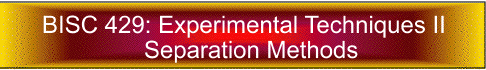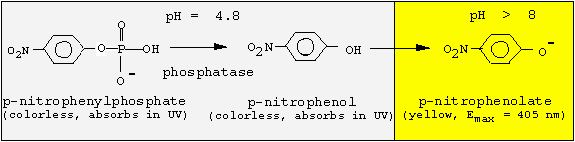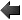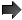## Acid phosphatase - Enzyme assay

Introduction
Results

Acid phosphatase hydrolyses phosphate ester bonds under an optimum pH of 4.8 and an optimum temperature of 37 °C. In this experiment you will use an artificial substrate, para-nitrophenylphosphate which will be hydrolyzed by acid phosphatase, releasing p-nitrophenol and inorganic phosphate (Pi).Both para-nitrophenylphosphate and p-nitrophenol are colorless at acidic pH values. Addition of 0.05 M NaOH after 30 min incubation at 37 °C will stop the reaction due to an increase in the pH of the reaction medium. Para-nitrophenol is yellow at alkaline pH and its concentration can be measured at 405 nm. Verify the results using Beer's law:

E=c d= 16,900 l/mol.cm at Emax = 405 nm; c = concentration [mol/l]; d = path length = distance between sides of cuvette = 1 cm.

### Experimental protocol

1. You will use known amounts of the reaction product p-nitrophenol (in citrate buffer) to obtain a standard curve. The stock solution provided is 10 µmol/ml. As p-nitrophenol is a skin irritant, you should wear gloves throughout these assays.

2. Prepare a sub-stock by diluting 100 µl of original (10 µmol/ml) to 2000 µl using citrate buffer (pH 4.8). Calculate and record the concentration of the substock. Also calculate the amount of p-nitrophenol in each vial and complete the 2nd column in Table 3.

3. Pipette the volumes given in Table 3, and measure and record the absorbance at 405 nm in the spectrophotometer. Use a pair of plastic cuvettes, and start with the most dilute sample.

4. Prepare the substrate solution (3 tablets = 15 mg p-nitrophenylphosphate in 10 ml citrate buffer, = 5.5 mM). 5. Now you have to determine enzyme activities in all the original aliquots and for the fractions obtained from gel filtration. For each of your aliquots, use 50 µl each of both undiluted and 1:10 diluted sample. For the fractions from gel filtration which tend to be very dilute, use 50 µl of undiluted sample.

6. Using appropriate pipetman, pipette each sample and buffer in to clean test tubes (see Table 4).

7. Add the substrate and start timing. Vortex and leave at room temperature for 30 min.

8. Add 2 ml of 0.05 M NaOH.

9. Measure absorbance at 405 nm. Use the same plastic cuvette for all samples.

One unit of enzyme (u) is defined as the the amount of enzyme that will produce 1 nmol of p-nitrophenol per minute.

### Purification table

The purpose of a purification table is to monitor the progress of the enzyme purification. Both yield and relative purity of the enzyme are calculated, taking advantage of protein concentration and enzyme activity experimentally determined for each fraction.

First we must transfer the data from table 3 and 4 into the purification table. Make sure to use the values of protein and enzyme concentration for each original, undiluted fraction; also note the total volume of the fractions.

For each fraction, divide the enzyme concentration by the protein concentration:

enzyme concentration [u/ml]
______________________ = specific activity [u/mg]
protein concentration [mg/ml]

The specific activity indicates how much of the total protein is the enzyme we try to isolate; therefore, its value should increase as the purification progresses. A purification step where the specific activity goes down would be counterproductive; the enzyme would be less pure than before.

A measure for the relative purity of a fraction (x) is the purification factor; it is defined as:

specific activity of (x)
______________________________ = purification factor for (x)
specific activity of starting material

Per definition, the purification factor for the starting material (the initial homogenate HOM) equals 1; increasing values indicate increasing purity. The degree of purity needed depends on the ultimate use of the isolated enzyme; normally, you would strive to purify an enzyme to "homogeneity", which means that you isolate the enzyme free of any other contaminating protein. The actual value of the purification factor varies widely, since it depends on the ratio between enzyme and other proteins in the starting material. If the protein has been purified to homogeneity, additional separation steps should not change the specific activity.

In practical terms, not only the purity of the enzyme matters, but also how much of the enzyme you recover. For each fraction (x), this is expressed as the yield:

total enzyme activity of fraction (x)
__________________________________ = yield
total enzyme activity in starting material

The yield in the homogenate is defined as 100 %; each purification step will result in some loss of enzyme activity, hence the yield is always less than 100 %. For each purification step, one always must achieve a balance between high yield and highest increase in purity. For example, if you only have a 2 mL fraction of the gel filtration column, you would probably get the highest purity of phosphatase; however, this would not be economical because you would have to run the column several times to get an acceptable yield.

Now complete the purification table and calculate the specific activities and yield; evaluate each purification step, and make suggestions for improvements. The progress of protein purification can also be monitored by gel electrophoresis; a pure protein should yield a single band in a native (=non-denaturating) gel.[ BISC 429 home] [ Enzyme isolation ] [ Lipoprotein isolation ] [ Lipid analysis ] [ DNA isolation ] [ feedback ]